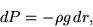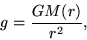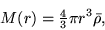Numerical Simulation

of a Hydrostatic Equation

Collapsing Nebula Cloud

In this numerical simulation we examine the same nebula cloud with the mass of 50 Suns and a diameter of 1 light year that is examined in a brief of an article below and compare results.

http://www.space.com/scienceastronomy/astronomy/stellar_chaos_020424.html

The findings are the result of computer simulations led by Matthew Bate of the University of Exeter and which were presented in early April at the UK National Astronomy Meeting.

The simulation followed the collapse of an interstellar gas cloud more than 1 light-year in diameter and containing a mass of gas and dust equal to 50 Suns. The end result, after 266,000 years, was the formation of a cluster of stars typical to our galaxy.

Yet of the 50 objects that were formed, only about half became stars. The rest were gravitationally ejected from the central cluster before they gathered enough mass to trigger thermonuclear fusion, the fusing of hydrogen into helium that powers a real star.

The ostracized failures are considered brown dwarfs.

Darwinian Star Formation

Here we examine the step by step process of nebula cloud condensation and the hydrostatic relationship between the reduction radius, increase of pressure within the spherical nebula cloud and does gravitational acceleration factor in to provide an equilibrium.

Formulas:

Gravitational Acceleration = G *M/ R2

standard formulas for Volume of a sphere & pressure

A spherical homogenous body in hydrostatic equilibrium satisfies the hydrostatic equation, sowhere p is the density and dP is the pressure change over a distance dr. But the gravitational acceleration is given bywhere G is the gravitational constant and the mass interior to radius r byConstants:

G = 6.67e-11

M = 50*1.989e30

Breaking the equation down to a simplified set of steps where the radius is reduced by a factor of 10 from the initial 1 light year diameter, a pattern emerges. Pressure within the cloud has a mean increase at a factor of 1000 times the initial, but the initial stellar mass (50 times that of our Sun) now compressed into a cloud with a radius .1 of original. This generates gravitational acceleration at a factor of 100 times the initial, thus the factor 10 problem surfaces. For any nebula cloud and its set initial pressure, a reduction of its radius by a factor of 10 increases the ratio between gravitational acceleration to pressure increase by a factor of 10. The relationship compounds for each succeeding reduction of radius by a factor of 10. Examples of consecutive factor 10 step reductions in the radius is listed below in the table. What is amazing is the mass of 50 suns contained within spherical nebula cloud a light year in diameter only produced a gravitational acceleration at the shell of the cloud of only 2.96 * 10-12 meters/ sec2 and this force is suppose to initiate collapse, how? If the cloud was allowed to shrink to 1/100,000 of its original size, we can see gravitational acceleration to pressure increase is 100,000 times greater than initial yet the cloud only produces a gravitational acceleration of 2.96 meters/sec2. A resultant less than a third of what occurs on the surface of the Earth while the cloud's radius is 23 million km with all its mass of 50 suns would be contained in less than half the orbit of Mercury. How would star formation occur in a cloud such as this? Any spark of a star would blow out the nebula cloud with nuclear expansion a solar wind. The conclusion here is that a hydrogen- helium nebula cloud could not collapse due to its own self contained mass due the factor 10 problem of the hydrostatic equation.

 Radius in Light Years Increase in Pressure P = Initial Gravitational Acceleration m/sec2 .5 P 2.96e-10 .05 1e3 P 2.96e-8 .005 1e6 P 2.96e-6 .0005 1e9 P 2.96e-4 .00005 1e12 P 2.96e-2 .000005 1e15 P 2.96

How can we equate the force of Pressure to the basic formula P= M/V when this is related to density?

Ideal Gas Law  PV = nRT is the true formula for gas in a nebula cloud, but how would this equation affect total results?

• n = number of moles
• R = universal gas constant = 8.3145 J/mol K
• T = Temperature

The relationship between shrinkage of the of the spherical cloud  and a reduction of its radius to .1 of its original size to an increase the variable of P by a factor of 1000 is not dependent upon the constant R or number of moles, which is related to mass and moles/gram in the Ideal Gas Law. Mankind must realize that the fault with theories related to the collapsing nebula cloud, which constitutes the basis for stellar formation and planetary formation has a fault, the factor 10. Independent of initial pressures and gravitational acceleration due to total mass of the cloud, the ratio of factor 10 between gravitational acceleration and pressure remains the same for all random combinations of nebula clouds for each transition step. If on was to take the derivative of and equation where the ratio was  x2 :: x3 with a constant, would not the constant go to 0 and the ratio be reduced to a factor 10? It is time to consider alternate to theory pertaining to the birth of stellar objects.

In the equation below

P = nRT/V

where V is a sphere if the radius is reduced to .1r, then P in this equation no matter what value it has, is a 1000 times greater so for all values of nRT the defined ratio of P to Pint is 1000P = nRT/V when the r subcomponent of V is reduced to .1r.

# Facts

• 1 Light Year = 9.4605284 × 1015 meters
• 2.365e7 km. = .000005 Light Year
• Mean Orbital Distance of Mercury 5.7e7 km.
• Gravitational Acceleration on Earth 9.78 m/sec2

## Parameters

4.433481e47 m3 =  initial Volume

Spherical Shape for the nebula cloud

Density variable but total mass always equals 50 Suns for given volume

Temperature near 00 Kelvin thus a constant (If Temperature becomes a factor it increases the pressure negating a percentage of gravitational acceleration)

Mean P = 50*1.989e30 kg./ initial V

I would truly like to thank God for bestowing this knowledge